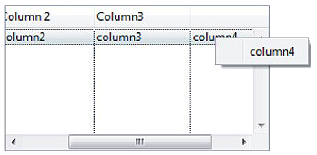Omnis Technical Note TNGI0029 Updated June 2012

for Omnis Studio 5 or above
by Götz Krija

The Headed List Box does not provide any hint about on which column a user has clicked. So what can you do when you want to display a context menu which should be different depending on the different columns of the object?

To solve this problem you can use the mouseover() function. You can determine the horizontal position of the click within the Headed List Box by the difference between the value provided by the mouseover() function and the position of the Headed List Box within the window. If you then add the scroll units to this value you will receive the exact position of the click even when the user has scrolled to the right within the Headed List Box. One scroll unit equates 8 pixels. This results in the following calculation:

Calculate horzPos as (mouseover(kMHorz)-\$cobj.\$left)+(\$cobj.\$hscroll-1)*8

Using \$columnwidths you can calculate the column being clicked on. This property provides a list with the width value for each column, each separated by comma. When you add the single column widths in a loop until the total gets bigger than the earlier calculated horizontal position of the click, the specific column number is the result of the number of loops required.

Calculate columnWidths as \$cobj.\$columnwidths
Repeat
Calculate colNum as colNum+1
Calculate colRight as colRight+strtok('columnWidths',",")
Until colRight>horzPos

In order to build a context menu dynamically you need an empty menu which you should deposit in the \$contextmenu property of the Headed List box. You need to add an \$init() method in the menu class in which the different menu lines, methods and the method text will be added at runtime:

; \$init method in menu class 'm1'
; create the vars: pColNum (Long integer), menuLineRef (Item reference)
; methodLineRef (Item reference)
Calculate methodLineRef.\$text as 'OK message {do something}'

You can call this method from within the Headed List Box and pass the column number as a parameter:

The result will look like this:The complete code behind the Headed List Box is the following:

; '\$event' method
; create the vars: colNum (Long integer), colRight (Long integer)
; columnWidths (Character), horzPos (Long integer)
Calculate hrzPos as (mouseover(kMHorz)-\$cobj.\$left)+(\$cobj.\$hscroll-1)*8
Calculate columnWidths as \$cobj.\$columnwidths
Repeat
Calculate colNum as colNum+1
Calculate colRight as colRight+strtok('columnWidths',",")
Until colRight>horzPos

If you change the \$columnsvisible for the headed list, then the above code will not work. You will need to add code to watch for settings in \$columnsvisible; see the sample library.

; '\$event' method
; create the following Local Variables
; colNum Long integer
; colRight Long integer
; colsVisible Character 100000000
; columnWidths Character 100000000
; hrzPos Long integer
; visible Boolean
Calculate hrzPos as (mouseover(kMHorz)-\$cobj.\$left)+(\$cobj.\$hscroll-1)*8
Calculate columnWidths as \$cobj.\$columnwidths
Calculate colsVisible as \$cobj.\$columnsvisible
Repeat
Calculate colNum as colNum+1
If strtok('colsVisible',",")
Calculate colRight as colRight+strtok('columnWidths',",")
Calculate visible as kTrue
Else
Do strtok('columnWidths',",")
Calculate visible as kFalse
End If
Until colRight>hrzPos|isclear(colsVisible)
If not(visible) ;; last column is not visible
Calculate colNum as colNum-1
End If